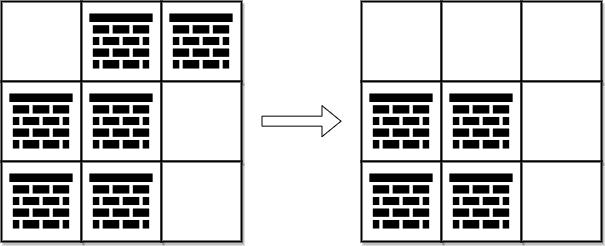##### Welcome to Subscribe On Youtube

Formatted question description: https://leetcode.ca/all/2290.html

# 2290. Minimum Obstacle Removal to Reach Corner

• Difficulty: Hard.
• Related Topics: Array, Breadth-First Search, Graph, Heap (Priority Queue), Matrix, Shortest Path.
• Similar Questions: Shortest Path in a Grid with Obstacles Elimination.

## Problem

You are given a 0-indexed 2D integer array grid of size m x n. Each cell has one of two values:

• 0 represents an empty cell,

• 1 represents an obstacle that may be removed.

You can move up, down, left, or right from and to an empty cell.

Return the **minimum number of obstacles to remove so you can move from the upper left corner (0, 0) to the lower right corner **(m - 1, n - 1).

Example 1:Input: grid = [[0,1,1],[1,1,0],[1,1,0]]
Output: 2
Explanation: We can remove the obstacles at (0, 1) and (0, 2) to create a path from (0, 0) to (2, 2).
It can be shown that we need to remove at least 2 obstacles, so we return 2.
Note that there may be other ways to remove 2 obstacles to create a path.


Example 2:Input: grid = [[0,1,0,0,0],[0,1,0,1,0],[0,0,0,1,0]]
Output: 0
Explanation: We can move from (0, 0) to (2, 4) without removing any obstacles, so we return 0.


Constraints:

• m == grid.length

• n == grid[i].length

• 1 <= m, n <= 105

• 2 <= m * n <= 105

• grid[i][j] is either 0 or 1.

• grid == grid[m - 1][n - 1] == 0

## Solution

• class Solution {
public int minimumObstacles(int[][] grid) {
int n = grid.length;
int m = grid.length;
int[][] dirs = new int[][] { {0, 1}, {1, 0}, {0, -1}, {-1, 0} };
Queue<State> q = new PriorityQueue<>((a, b) -> a.removed - b.removed);
boolean[][] visited = new boolean[n][m];
visited = true;
while (!q.isEmpty()) {
State state = q.poll();
if (state.r == n - 1 && state.c == m - 1) {
return state.removed;
}
for (int[] d : dirs) {
int nr = state.r + d;
int nc = state.c + d;
if (nr < 0 || nc < 0 || nr == n || nc == m || visited[nr][nc]) {
continue;
}
visited[nr][nc] = true;
State next = new State(nr, nc, state.removed);
if (grid[nr][nc] == 1) {
next.removed++;
}
}
}
return -1;
}

private static class State {
int r;
int c;
int removed;

State(int r, int c, int removed) {
this.r = r;
this.c = c;
this.removed = removed;
}
}
}

• Todo

• class Solution:
def minimumObstacles(self, grid: List[List[int]]) -> int:
m, n = len(grid), len(grid)
q = deque([(0, 0, 0)])
vis = set()
dirs = (-1, 0, 1, 0, -1)
while 1:
i, j, k = q.popleft()
if i == m - 1 and j == n - 1:
return k
if (i, j) in vis:
continue
for a, b in pairwise(dirs):
x, y = i + a, j + b
if 0 <= x < m and 0 <= y < n:
if grid[x][y] == 0:
q.appendleft((x, y, k))
else:
q.append((x, y, k + 1))

############

# 2290. Minimum Obstacle Removal to Reach Corner
# https://leetcode.com/problems/minimum-obstacle-removal-to-reach-corner

class Solution:
def minimumObstacles(self, grid: List[List[int]]) -> int:
rows, cols = len(grid), len(grid)
​
dist = [[float('inf')] * cols for _ in range(rows)]
dist = 0

heap = [(0, 0, 0)]

while heap:
k, x, y = heappop(heap)

if dist[x][y] != k:
continue

for dx, dy in [(x + 1, y), (x - 1, y), (x, y + 1), (x, y - 1)]:
if 0 <= dx < rows and 0 <= dy < cols:
old = dist[dx][dy]
new = k + grid[dx][dy]

if new < old:
dist[dx][dy] = new
heappush(heap, (new, dx, dy))

return dist[-1][-1]



Explain:

nope.

Complexity:

• Time complexity : O(n).
• Space complexity : O(n).